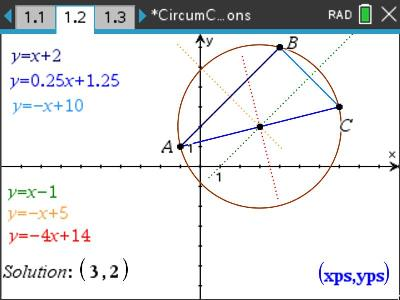# Activities

•• ##### AuthorAust Senior

50 Minutes

• ##### Device
•TI-Nspire™ CX
•TI-Nspire™ CX CAS
• ##### Software

TI-Nspire™
TI-Nspire™ CAS

4.5

## Circumcircles#### Activity Overview

A circumcircle is a unique circle that passes through all three of a triangle's vertices. In this activity students start with the geometrical entity and then transfer this to the Cartesian plane where they determine equations to lines (given two points), equations to lines (given point and gradient), intersection of two lines and finally the equation to a circle. Once students have completed the prescribed points they are required to come up with their own three points, a TI-nspire teacher file generates all the required equations given three starting points.

#### Objectives

Students are required to to determine the following:

• Equation to a straight line given two points.
• Equation to a straight line given the gradient (perpendicular) and a point.
• Point of intersection between two or more lines.
• Equation to a circle given centre and radius

#### Vocabulary

• Circumcentre
• Circumcircle
• Perpendicular bisector
• Simultaneous equations